# A Isosurface as least squares minimizer

It is possible to show that the isosurface of the weighted signed distance function is equivalent to a least squares minimization of squared distances between points on the range surfaces and points on the desired reconstruction. The key assumptions are that the range sensor is orthographic and that the range errors are independently distributed along sensor lines of sight. A full proof is beyond the scope of this paper, but we provide a sketch here. See  for details.

Consider a region, R, on the desired surface, f, which is observed by n range images. We define the error between an observed range surface and a possible reconstructed surface as the integral of the weighted squared distances between points on the range surface and the reconstructed surface. These distances are taken along the lines of sight of the sensor, commensurate with the predominant directions of uncertainty (see Figure 12). The total error is the sum of the integrals for the n range images: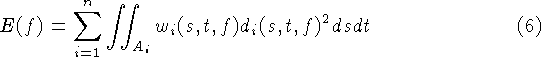where each (s, t) corresponds to a particular sensor line of sight for each range image,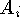is the domain of integration for the i'th range image, and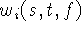and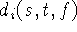are the weights and signed distances taken along the i'th range image's lines of sight.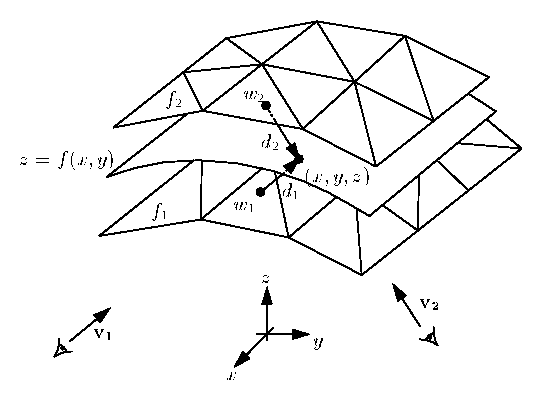Figure 12: Two range surfaces,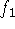and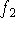, are tessellated range images acquired from directions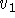and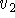. The possible range surface, z = f(x,y), is evaluated in terms of the weighted squared distances to points on the range surfaces taken along the lines of sight to the sensor. A point, (x,y,z), is shown here being evaluated to find its corresponding signed distances,and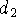, and weights,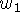and.

Now, consider a canonical domain, A, on a parameter plane, (x,y), over which R is a function z = f(x,y). The total error can be re-written as an integration over the canonical domain: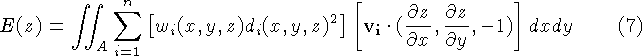where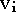is the sensing direction of the i'th range image, and the weights and distances are evaluated at each point, (x,y,z), by first mapping them to the lines of sight of the corresponding range image. The dot product represents a correction term that relates differential areas in A to differential areas in. Applying the calculus of variations , we can construct a partial differential equation for the z that minimizes this integral. Solving this equation we arrive at the following relation: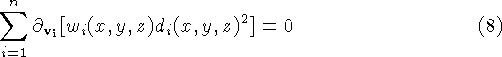where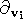is the directional derivative along. Since the weight associated with a line of sight does not vary along that line of sight, and the signed distance has a derivative of unity along the line of sight, we can simplify this equation to: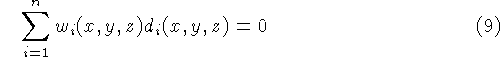This weighted sum of signed distances is the same as what we compute in equations 1 and 2, without the division by the sum of the weights. Since the this divisor is always positive, the isosurface we extract in section 3 is exactly the least squares minimizing surface described here.